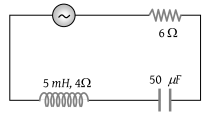• Subject:

...

• Topic:

...

In the circuit shown below, the ac source has voltage $V=20\mathrm{cos}\left(\omega t\right)$ volts with ω = 2000 rad/sec. the amplitude of the current will be nearest to(1) 2A

(2) 3.3 A

(3) $2/\sqrt{5}A$

(4) $\sqrt{5}A$